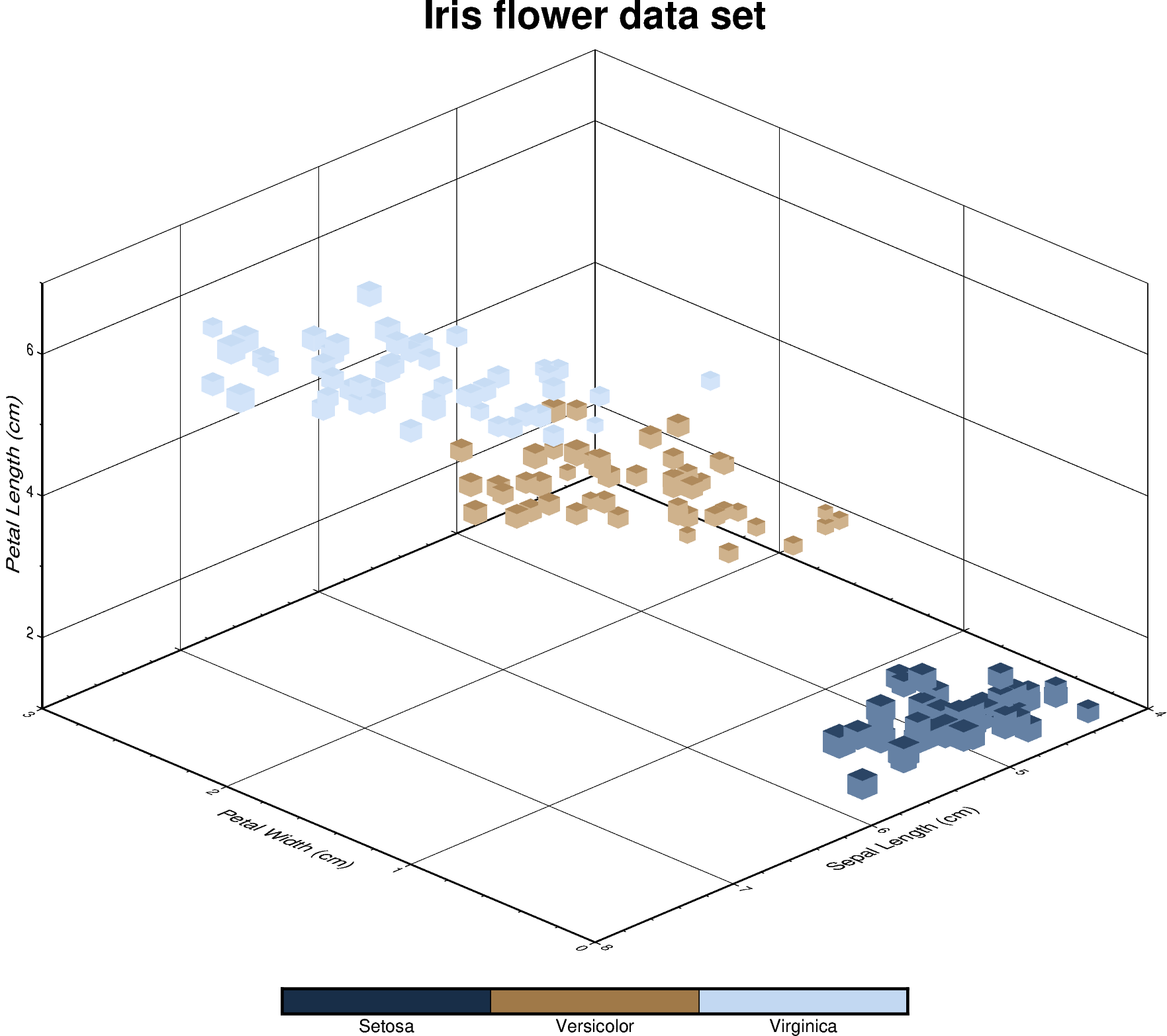# 3D Scatter plots¶

The pygmt.Figure.plot3d method can be used to plot symbols in 3D. In the example below, we show how the Iris flower dataset can be visualized using a perspective 3D plot. The region parameter has to include the $$x$$, $$y$$, $$z$$ axis limits in the form of (xmin, xmax, ymin, ymax, zmin, zmax), which can be done automatically using pygmt.info. To plot the z-axis frame, set frame as a minimum to something like frame=["WsNeZ", "zaf"]. Use perspective to control the azimuth and elevation angle of the view, and zscale to adjust the vertical exaggeration factor.Out:

<IPython.core.display.Image object>


import pandas as pd
import pygmt

# Load sample iris data and convert 'species' column to categorical dtype
df.species = df.species.astype(dtype="category")

# Use pygmt.info to get region bounds (xmin, xmax, ymin, ymax, zmin, zmax)
# The below example will return a numpy array like [0.0, 3.0, 4.0, 8.0, 1.0, 7.0]
region = pygmt.info(
table=df[["petal_width", "sepal_length", "petal_length"]],  # x, y, z columns
per_column=True,  # report the min/max values per column as a numpy array
# round the min/max values of the first three columns to the nearest multiple
# of 1, 2 and 0.5, respectively
spacing=(1, 2, 0.5),
)

# Make a 3D scatter plot, coloring each of the 3 species differently
fig = pygmt.Figure()

# Define a colormap to be used for three categories, define the range of the
# new discrete CPT using series=(lowest_value, highest_value, interval),
# use color_model="+cSetosa,Versicolor,Virginica" to write the discrete color palette
# "cubhelix" in categorical format and add the species names as annotations for the
# colorbar
pygmt.makecpt(
cmap="cubhelix", color_model="+cSetosa,Versicolor,Virginica", series=(0, 2, 1)
)

fig.plot3d(
# Use petal width, sepal length and petal length as x, y and z data input,
# respectively
x=df.petal_width,
y=df.sepal_length,
z=df.petal_length,
# Vary each symbol size according to another feature (sepal width, scaled by 0.1)
size=0.1 * df.sepal_width,
# Use 3D cubes ("u") as symbols, with size in centimeter units ("c")
style="uc",
# Points colored by categorical number code
color=df.species.cat.codes.astype(int),
# Use colormap created by makecpt
cmap=True,
# Set map dimensions (xmin, xmax, ymin, ymax, zmin, zmax)
region=region,
# Set frame parameters
frame=[
'WsNeZ3+t"Iris flower data set"',  # z axis label positioned on 3rd corner, add title
'xafg+l"Petal Width (cm)"',
'yafg+l"Sepal Length (cm)"',
'zafg+l"Petal Length (cm)"',
],
# Set perspective to azimuth NorthWest (315°), at elevation 25°
perspective=[315, 25],
# Vertical exaggeration factor
zscale=1.5,
)